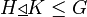# Join lemma for normal subgroup of subgroup with normal subgroup of whole group

## Statement

### Statement with symbols

If$H \underline{\triangleleft} K \le G$ and$L \underline{\triangleleft} G$, then$\langle H, L \rangle \underline{\triangleleft} \langle K, L \rangle$.